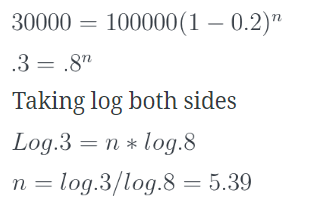Courses

# A machine is depreciated at the rate of 20% on reducing balance. The original cost of the machine was Rs. 100000 and its ultimate scrap value was Rs. 30000. The effective life of the machine isa)4.5 years (appx.)b)5.4 years (appx.)c)5 years (appx.)d)none of theseCorrect answer is option 'B'. Can you explain this answer? Related Test: Test: Simple And Compound Interest Including Annuity - 1

## CA Foundation QuestionFsgssg Yidxd Feb 07, 2019
How to find log.3 & log.8Sagarika Shinde Aug 28, 2018
Net worth (value)=initial value *(1-i)^n
Where, I is the rate of depreciation/100 and n is the number of conversion period
I=0.2
Fv=30000, initial value=100000Ila Desai Oct 28, 2018
4.5Dhanusha Thamizh Sep 10, 2019
How